• 怎么对比两张图片
千次阅读
2018-12-06 20:18:27

haclon源码

read_image (Image, 'C:/Users/bayinglong/Desktop/搜狗截图20181206101404.png')
rgb1_to_gray (Image, GrayImage1)
rgb1_to_gray (Image1, GrayImage2)
abs_diff_image ( GrayImage1,  GrayImage2, ImageAbsDiff, 1)
a:=0
get_image_size (ImageAbsDiff, Width, Height)
gen_rectangle1 (Rectangle, 1,1,Height,Width)
intensity (Rectangle, ImageAbsDiff, Mean, Deviation)



主要流程，
1.两张图片相减少
2.相减图片求平均值和方差；

c#核心代码

public bool Extcute(out double Deviation, HImage bitmap1, HImage bitmap2)
{
try
{
ho_GrayImage1.Dispose();
HOperatorSet.Rgb1ToGray(bitmap1, out ho_GrayImage1);
ho_GrayImage2.Dispose();
HOperatorSet.Rgb1ToGray(bitmap2, out ho_GrayImage2);
ho_ImageAbsDiff.Dispose();
HOperatorSet.AbsDiffImage(ho_GrayImage1, ho_GrayImage2, out ho_ImageAbsDiff, 1);
HOperatorSet.GetImageSize(ho_ImageAbsDiff, out hv_Width, out hv_Height);
ho_Rectangle.Dispose();
HOperatorSet.GenRectangle1(out ho_Rectangle, 1, 1, hv_Height, hv_Width);
HOperatorSet.Intensity(ho_Rectangle, ho_ImageAbsDiff, out hv_Mean, out hv_Deviation);

Deviation = (double)hv_Deviation;
return true;
}
catch (System.Exception ex)
{
Deviation = 1000;
return false;
}

}


利用图像句柄生成haclon Himage类

 /// <summary>
/// 生成Himage
/// </summary>
/// <param name="bmp">图片句柄</param>
/// <param name="width">图片宽</param>
/// <param name="hight">图片高</param>
/// <returns></returns>
public HImage HImageConvertFromBitmap32(IntPtr bmp,int width,int hight)
{

HalconDotNet.HImage img = new HalconDotNet.HImage();
img.GenImage1("byte", width, hight, bmp);
return img;
}

更多相关内容
• 主要介绍了用python实现对比两张图片的不同的相关资料,需要的朋友可以参考下
• print(lst_data) print(min(lst_data)) print(max(lst_data)) 图片1： shape:(26, 86) 图片2： shape:(25, 85) 两张图片大小差一个像素，对比结果为 0.9991 2. SSIM（结构相似性度量） 这是一种全参考的图像质量评价...

# 1.cosin相似度（余弦相似度）

from PIL import Image
from numpy import average, linalg, dot

def get_thumbnail(image, size=(30, 30), greyscale=False):
image = image.resize(size, Image.ANTIALIAS)
if greyscale:
image = image.convert('L')
return image

def image_similarity_vectors_via_numpy(image1, image2):
image1 = get_thumbnail(image1)
image2 = get_thumbnail(image2)
images = [image1, image2]
vectors = []
norms = []
for image in images:
vector = []
for pixel_tuple in image.getdata():
vector.append(average(pixel_tuple))
vectors.append(vector)
norms.append(linalg.norm(vector, 2))
a, b = vectors
a_norm, b_norm = norms
res = dot(a / a_norm, b / b_norm)
return res

import os
path = './duibi/凭证/'
path2 = './duibi/印泥/'
files2 = os.listdir(path2)
# print(files2)
lst_data = []
for root, dirs, files in os.walk(path):
for file in files:
image1 = Image.open(os.path.join(root, file))
for f in files2:
image2 = Image.open(os.path.join(path2, f))
cosin = image_similarity_vectors_via_numpy(image1, image2)
lst_data.append(cosin)
# print(cosin)
print(lst_data)
print(min(lst_data))
print(max(lst_data))


图片1：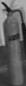shape:(26, 86)
图片2：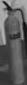shape:(25, 85)
两张图片大小差一个像素，对比结果为 0.9991

# 2. SSIM（结构相似性度量）

这是一种全参考的图像质量评价指标，分别从亮度、对比度、结构三个方面度量图像相似性。

SSIM取值范围[0, 1]，值越大，表示图像失真越小。

在实际应用中，可以利用滑动窗将图像分块，令分块总数为N，考虑到窗口形状对分块的影响，采用高斯加权计算每一窗口的均值、方差以及协方差，然后计算对应块的结构相似度SSIM，最后将平均值作为两图像的结构相似性度量，即平均结构相似性SSIM。

from skimage.measure import compare_ssim
import numpy as np

img2 = np.resize(img2, (img1.shape, img1.shape, img1.shape))

print(img2.shape)
print(img1.shape)
ssim = compare_ssim(img1, img2, multichannel=True)

print(ssim)


使用上面两张图片：结果为：0.146
看似结果比上面结果差很多

# 3. 基于直方图

直方图能够描述一幅图像中颜色的全局分布，是一种入门级的图像相似度计算方法。

from PIL import Image

def make_regalur_image(img, size = (256, 256)):
return img.resize(size).convert('RGB')

def hist_similar(lh, rh):
assert len(lh) == len(rh)
return sum(1 - (0 if l == r else float(abs(l - r))/max(l, r)) for l, r in zip(lh, rh))/len(lh)

def calc_similar(li, ri):
return hist_similar(li.histogram(), ri.histogram())

if __name__ == '__main__':
img1 = Image.open('1.jpg')
img1 = make_regalur_image(img1)
img2 = Image.open('2.jpg')
img2 = make_regalur_image(img2)
print(calc_similar(img1, img2))


结果为：0.845
直方图过于简单，只能捕捉颜色信息的相似性，捕捉不到更多的信息。只要颜色分布相似，就会判定二者相似度较高，显然不合理。

# 4. 基于互信息（Mutual Information）

通过计算两个图片的互信息来表征他们之间的相似度。

from sklearn import metrics as mr
import numpy as np

img2 = np.resize(img2, (img1.shape, img1.shape, img1.shape))

img1 = np.reshape(img1, -1)
img2 = np.reshape(img2, -1)
print(img2.shape)
print(img1.shape)
mutual_infor = mr.mutual_info_score(img1, img2)

print(mutual_infor)


结果：2.163
如果两张图片尺寸相同，还是能在一定程度上表征两张图片的相似性的。但是，大部分情况下图片的尺寸不相同，如果把两张图片尺寸调成相同的话，又会让原来很多的信息丢失，所以很难把握。经过实际验证，此种方法的确很难把握。

展开全文pytorch opencv python
• python 对比两张图片是否相同说明 说明 适用于对比两张图片是否完全相同 需要两张图片的大小完全一致 代码来源于网络，进行微调 from PIL import Image from PIL import ImageChops def compare_images(path_one...

# 说明

1. 适用于对比两张图片是否完全相同
2. 需要两张图片的大小完全一致

代码来源于网络，进行微调

from PIL import Image
from PIL import ImageChops

def compare_images(path_one, path_two):
"""
比较图片
:param path_one: 第一张图片的路径
:param path_two: 第二张图片的路径
:return: 相同返回 success
"""
image_one = Image.open(path_one)
image_two = Image.open(path_two)
try:
diff = ImageChops.difference(image_one, image_two)

if diff.getbbox() is None:
# 图片间没有任何不同则直接退出
return "success"
else:
return "ERROR: 匹配失败！"

except ValueError as e:
return "{0}\n{1}".format(e, "图片大小和box对应的宽度不一致!")

if __name__ == '__main__':
compare_images(
'target.png',
'template.jpg',
)


展开全文python
• 源码使用种方法实现图片的比较。一种是使用画板的取点颜色值对比，另一种是将图片数据放入内存中，对比内存字节集数据。
• 图片的存储、管理、查询、求解两张图片的相似度（基于颜色特征矩阵） 图片的存储、管理、查询、求解两张图片的相似度（基于颜色特征矩阵）
• color_image和data_initial是两张大小相同的图片 color_image==data_initial输出逐个像素比较的True和False 如： import numpy as np a = np.array([[1, 2, 3], [2, 3, 4]]) b = np.array([[1, 2, 3], [2, 2, 4]]) ...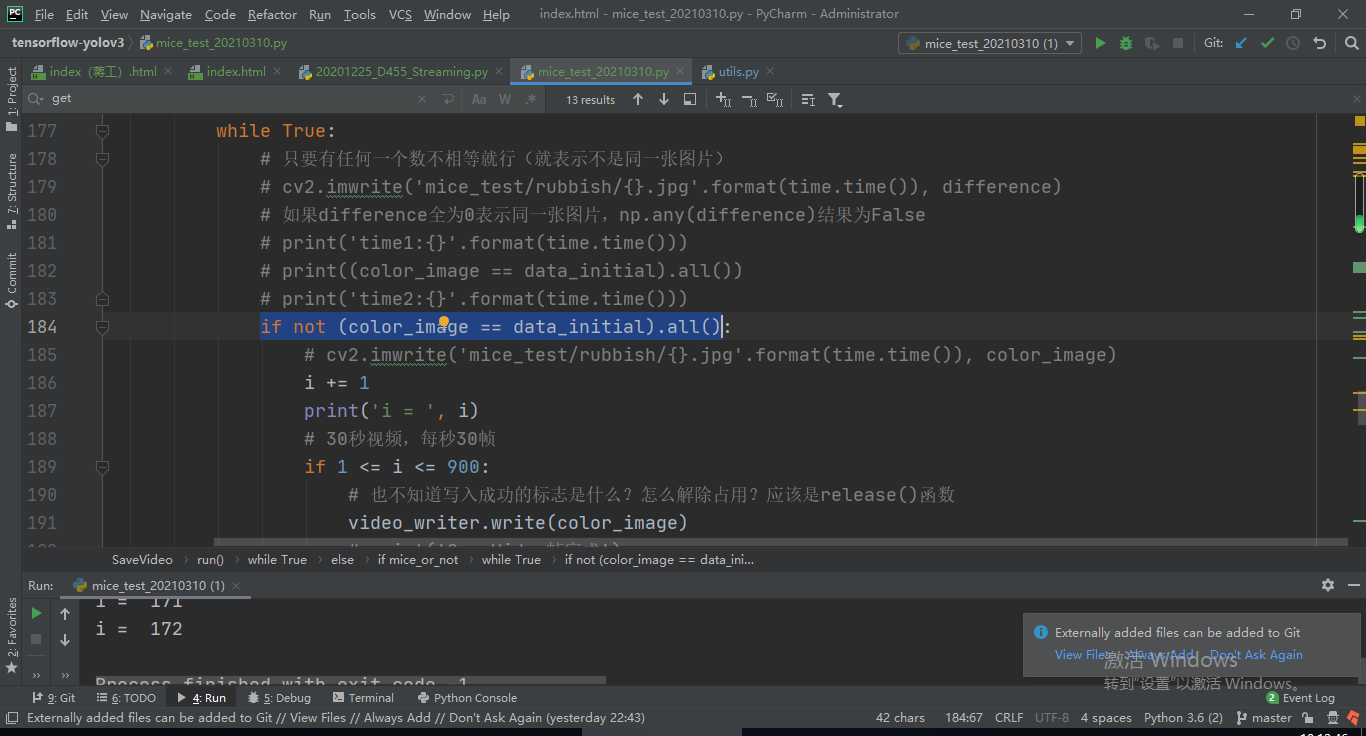用：

if not (color_image == data_initial).all():


color_image和data_initial是两张大小相同的图片

color_image==data_initial输出逐个像素比较的True和False
如：

import numpy as np

a = np.array([[1, 2, 3], [2, 3, 4]])
b = np.array([[1, 2, 3], [2, 2, 4]])
print(a == b)



输出结果：

[[ True  True  True]
[ True False  True]]


.all()方法就是如果数组里全是True，输出就为True，如果不全是True，输出就是False，这样就能判断两张图片是否相同了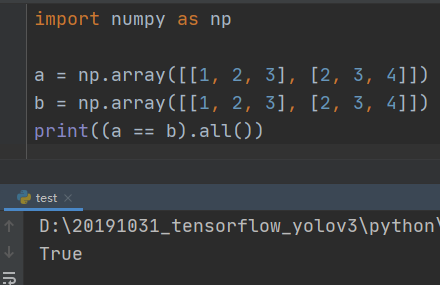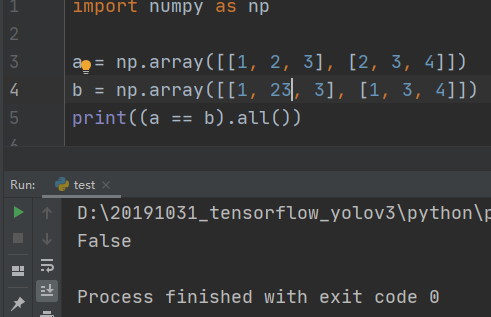展开全文• 比较两张图片：图片比较及截图代码，可以非常精确地发现图片是否一致，哪怕一个像素。可用于识别真伪，判断界面变化，行情到到账判断等。
• #Photos 比较脚本 如果你想使用这个脚本观看example/index.html 。JavaScript
• 图片上传的时候，通过对比两张图片的MD5值，判断图片是否上传成功。
• ## 用python对比两张图片的不同

万次阅读 多人点赞 2018-07-08 14:08:05
from PIL import Image from PIL import ImageChops def compare_images(path_one, path_two, diff_save_location): &quot;&quot;&quot;... 比较图片，如果有不同则... @参数二: path_two: 第二张图片的...
• 返回值是 'image1' 和 'image2'对比后的相似度，相似度越高，图片越接近，达到100.0说明图片完全相同。 ''' img1 = image1.resize(size).convert("RGB") sub_image1 = split_image(img1, part_size) img2 = ...
• import numpy import cv2 from PIL import Image def calculate(image1, image2): image1 = cv2....解决方法是循环对比5次，有一次大于80%就break退出循环，每一次对比睡眠1s，如果5次都对比失败了，则图片对比failpython 机器学习 计算机视觉
• 如何利用Python3和OpenCV对比两张图片的不同，提取差异性 导言：通过机器视觉来计算两个图片之间的差异性，可以快速有效辨别文件、图片是否被篡改，也能帮助用户轻松识别钓鱼网站，确保财产安全。 一、所需模块 pip ...python opencv 计算机视觉 人工智能 机器学习
• 这篇文章主要介绍了用python实现对比两张图片的不同的相关资料,需要的朋友可以参考下。 直接上代码如下： from PIL import Image import math import operator from functools import reduce def image_contrast...python 断言
• 下面代码计算了图片的平均亮度和亮度的标准差 思路：灰度就当作是亮度。平均亮度就是每个像素的灰度相加并除以总像素。标准差公式可百度…… 还有个图片的亮度，可参考...
• # 汉明距离表示个（相同长度）字对应位不同的数量，我们以 # d（x,y）表示个字x,y之间的汉明距离。对个字符串进行异或运算， # 并统计结果为1的个数，那么这个数就是汉明距离。 # 向量相似度越高，对应的汉明...python
• 判断两张图片的内容是否一致【Python 两种方法】马富天2019-12-12 17:42:2939【摘要】在很多时候需要对相同的图片进行去重处理，这些图片可能是格式不同（png、jpg等），但是图片的内容可能是一样的，我们需要对相同...
• 之前用按键精灵写过一些游戏辅助，里面有个函数叫FindPic，就上在屏幕范围查找给定的一张图片，返回查找到的坐标位置。现在，Java来实现这个函数类似的功能。算法描述：屏幕截图，得到图...进一步对比，将映射范围内...
• OpenCV识别出两张图片的人脸，并比较出两张人脸的相似度，并给出相似值。 包含完整的工程文件，可立即运行！！！ 用facedetect功能将两张图片中的人脸检测出来 将人脸部分的图片剪切出来，存到两张只有人脸的图片...人脸识别
• 本案例是利用Matlab来查找两幅图像的不同之处，利用SURF特征匹配的算法将图片对齐，之后采用形态学算法，对图像进行处理。大家下载之后可以快速的入门，欢迎大家下载。
• 1、原图上可以发现的信息，两张图拍摄...2、如果单从比较两张图片是否相同，那肯定不相同；若是比较图一中水泥模子是否为图二中水泥模子晾干之后的结果，那答案是相同的，可以将图一或者图二换成与之对应的相位就可..计算机视觉
• 一款简单实用的JS拖动滑块对比图片处理前后效果代码，通过拖动滑块来将原图和处理后的图片两张图片进行对比
• js人物头颅图片拖动对比特效是一款支持3张图片前后对比效果下载。
• 如何评估两张图片的差异 方法一，图像直方图比较 就像人的指纹一样，没有哪两张的照片的直方图会是一样的（拍摄的图片），所以只要将两张图片拉倒ps里面看直方图就可以了。 方法二，DiffImg 方法三，Image Comparer ......